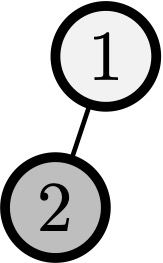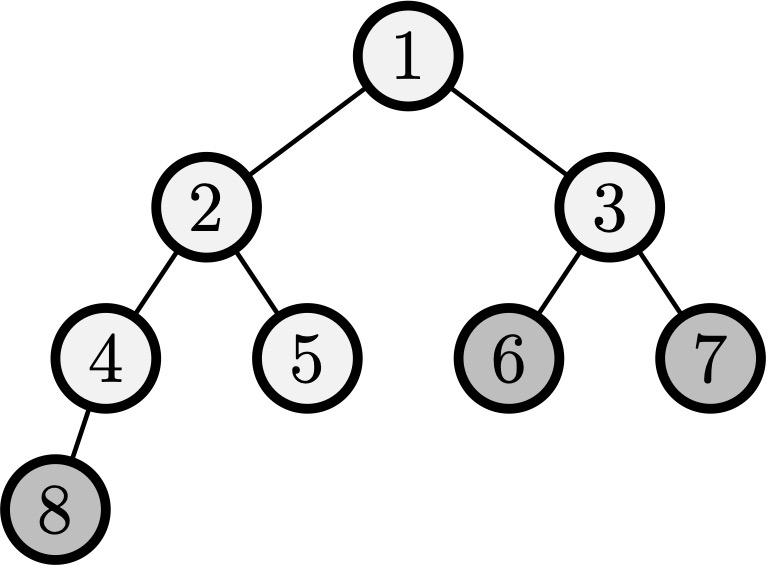시간 제한메모리 제한제출정답맞힌 사람정답 비율
2 초 (추가 시간 없음) 1024 MB0000.000%

## 문제

There is a coin tossing tournament organized by the Thieves Guild. A total of $x$ thieves and $y$ assassins are going to take part in the tournament. Initially, each participant has a position denoted by an integer from $1$ to $x + y$. The games happen while there are at least two participants. In each game, consider participant $A$ standing at the position with the greatest number. Let it be position $k$. Participant $A$ tosses a fair coin, hoping to move to position $\lfloor k / 2 \rfloor$ which is occupied by some participant $B$ at the moment. If $A$ got heads, then $A$ moves to $B$'s position, and $B$ is kicked out of the tournament. If $A$ got tails, then $A$ is kicked out of the tournament, and $B$ remains at the same position. The last remaining participant is the winner.

Делегация ассассинов опоздала к регистрации, так что воры заняли позиции от $1$ до $x$, и ассассинам остались позиции от $x + 1$ до $x + y$. Казначей турнира хочет заранее знать, какова вероятность победы ассассина на турнире, если во всех играх используется идеальная монетка, то есть вероятности выпадения <<орла>> и <<решки>> равны $1 / 2$ и не зависят друг от друга. Найдите эту вероятность.

The delegation of assassins was late for the registration, so the thieves already occupied the positions from $1$ to $x$, and the assassins were left with the positions from $x + 1$ to $x + y$. The tournament treasurer wants to know in advance what is the probability of an assassin winning the tournament, given that a fair coin is used for every game, that is, the probabilities of heads and tails are equal to $1 / 2$, and all coin tosses are independent. Find this probability.

## 입력

The first line contains two integers $x$ and $y$: the number of thieves and the number of assassins ($1 \le x, y \le 1\,000\,000$).

## 출력

Output the required probability as a decimal fraction. Your answer will be considered correct if the absolute or relative error will be less than $10^{-6}$.

## 예제 입력 1

1 1


## 예제 출력 1

0.5## 예제 입력 2

5 3


## 예제 출력 2

0.312500000000